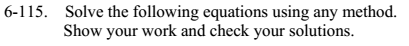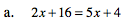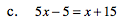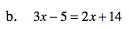### Home > MC2 > Chapter 6 > Lesson 6.2.5 > Problem6-115

6-115.Subtract 2x from both sides to move the variable onto one side of the equation.

2x + 16 = 5x + 4
− 2x − 2x
16 = 3x + 4

Isolate the variable by subtractingboth sides by 4.

16 = 3x + 4
−4 −4
12 = 3x

Finally, divide both sides by 3.

$x=\frac{12}{3}=4$Follow the method presented in part (a). Check your answering using the method presented in part (b).

Follow the method presented in part (a).

x = 19

Substitute 19 back into the equation.

3 (19) − 5 = 2 (19) + 14

Simplify.

57 − 5 = 38 + 14

52 = 52

If both sides of the equation are equal, your answer is correct.